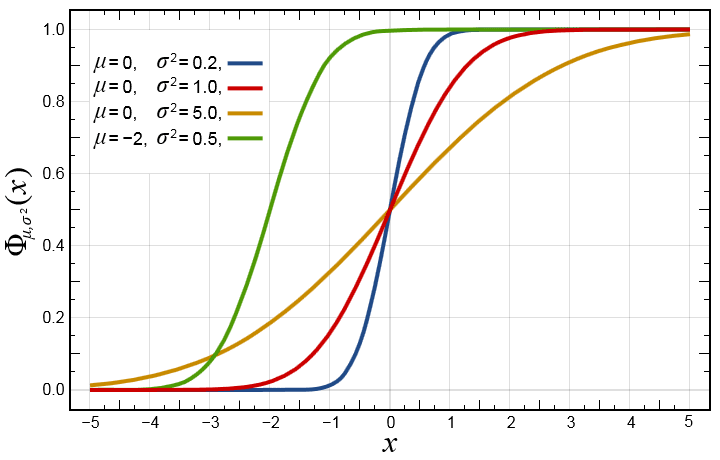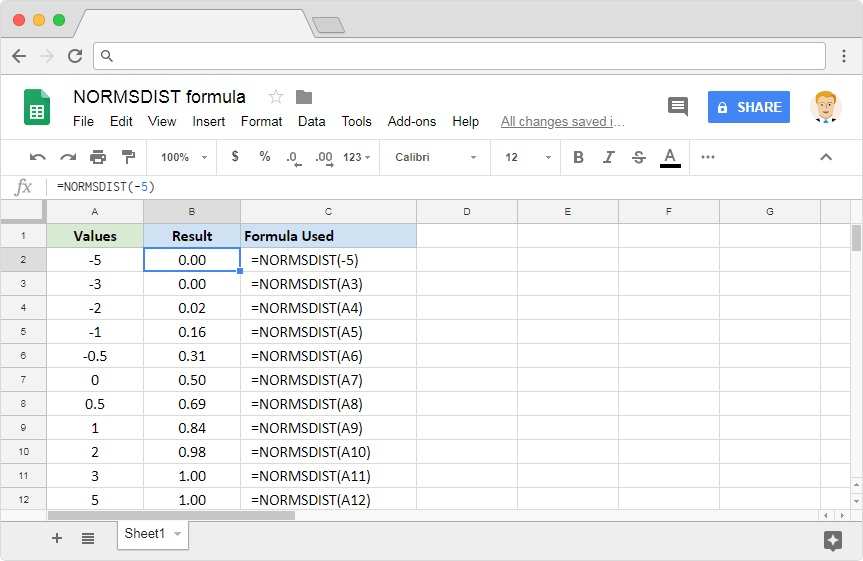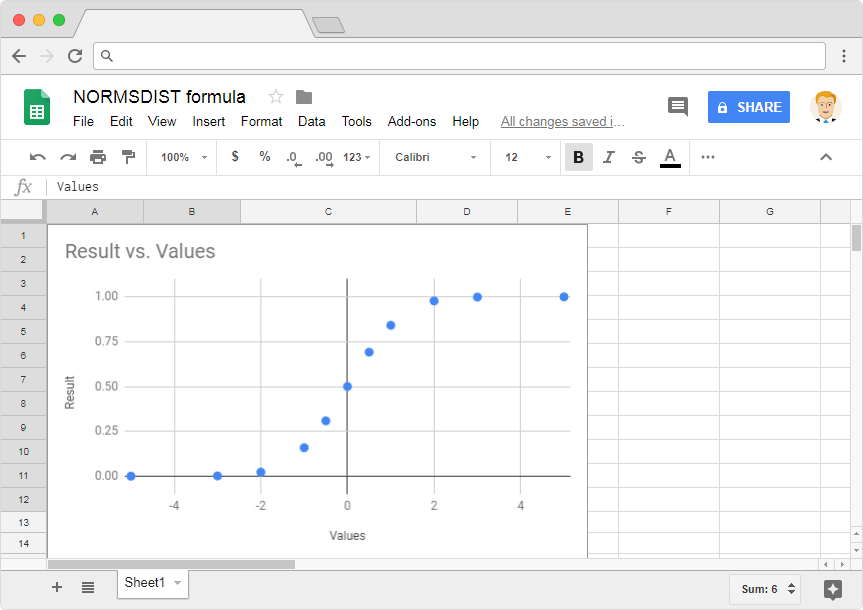# How to use the NORMSDIST formula in Google SheetsIn this post, learn how to calculate the normal cumulative distribution by using the NORMSDIST formulin Google Sheets. The normal cumulative distribution is one of the most widely used probability distributions in statistics. We can derive a specialized case of normal cumulative distribution when mean is zero and the variance is 1. This is called the standard normal cumulative distribution function. Here’s how the function looks like when we plot it on the graph – the red curve is what we have been talking about.Now, if we were to know the value of the standard normal cumulative distribution function against the normally distributed variable (x), we can use the NORMSDIST formula in Google Sheets.

### Syntax

NORMSDIST(x)

• x – is the input value or cell reference that we pass to the standard normal cumulative distribution function.

The formula has one of the simplest syntaxes in all of Google Sheets. All it takes is the value of one single parameter, and it returns the value of the standard normal cumulative distribution function corresponding to that input value.

### Usage: NORMSDIST formula in Google Sheets

Now that we learned the syntax, let us practically apply the formula in Google Sheets application. And we may compare the graph with that of the output values. Please have a look at the following snapshot.Like most other Google Sheets formulas, the NORMSDIST formula also is capable of accepting direct numeric values. And also references to the cells consisting of these numeric values. The first example has an input that is a direct numeric value. Whereas all other examples have cell references for its input.

Now let’s observe the output values the formula returned. If we made a quick comparison of the output values against those in the graph, it certainly looks the same. We can get a little creative and try to plot these output values on a graph using Google Sheets charts. And here’s how it looks like.Without a doubt, it does resemble the red curve graph shown in the very first image.

This is how you use the NORMSDIST formula in Google Sheets. Note that there is also a way to do the opposite. That is to find the value of the distributed variable, given the value of the distribution itself. The NORMSINV formula in Google Sheetshelps us achieve this calculation.